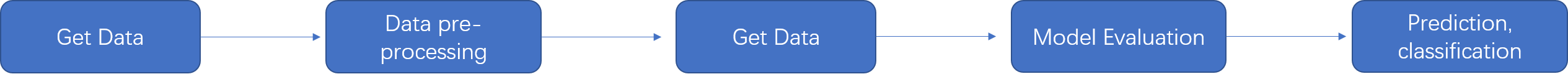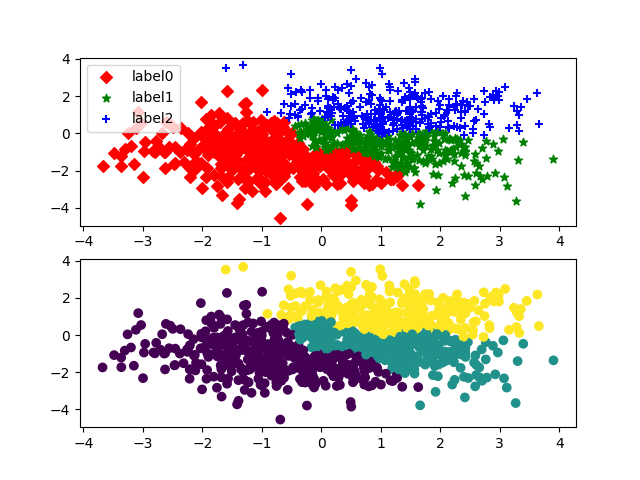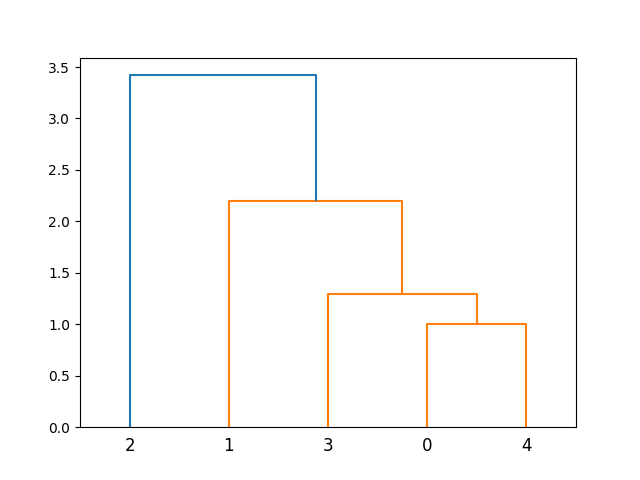rise

V1

2022/03/07阅读：26主题：红绯

# Python Clustering Analysis(I)

Machine learning is dedicated to the study of how to use experience to improve the performance of the system itself by means of computation.

Machine learning, as the main technique in big data analytics applications, has been widely used in various fields. Machine learning algorithms include two main categories, one is supervised learning algorithms and the other is unsupervised learning algorithms.

Cluster analysis is one of the unsupervised learning algorithms in machine learning algorithms.Cluster analysis is a statistical analysis technique that divides a set of research objects into relatively homogeneous groups.

The input of clustering is a set of unlabeled samples, and the clusters are divided into groups based on the distance or similarity of the data itself, with the principle of minimizing the intra-group distance and maximizing the inter-group (external) distance.

## Introduction to Machine learning library sklearn

The Python extension library sklearn is an open source library for data analysis and machine learning and machine learning that encapsulates common machine learning methods, including clustering, regression, dimensionality reduction, and classification.

The general process of machine learning is shown in the figure.## KMeans clustering algorithm

KMeans clustering algorithm is a simple and commonly used clustering algorithm, which belongs to unsupervised learning algorithm.In the initial state, the data samples have no labels or target values. The clustering algorithm discovers the relationship between the data samples, classifies the similar samples into one class, and labels them accordingly.

The purpose of the KMeans clustering algorithm is to classify each of the n y samples in the data set into k classes.

The basic idea of the KMeans clustering algorithm is to first select any k objects in the data set X as the initial cluster centers, and the remaining data objects are assigned to the nearest clusters according to their proximity to each cluster center.Then the average of the data in each of the assigned clusters is calculated and used as the center of the new cluster. The previous process is repeated until the cluster centers no longer change, ending the whole clustering process.

The general process of KMeans clustering algorithm is shown in the figure.

#### Usage of KMeans in sklearn.cluster

Common parameters of KMeans Class are as follows.

``n_clusters: int type, the number of clusters generated, default is 8.max_iter: int type, the maximum number of iterations performed by executing the k-means algorithm once. The default value is 300.n_init: int type, the number of times to run the algorithm with different initialization values of the cluster centers, the final solution is the best result selected in the inertia sense. The default value is 10.init: three optional values: 'k-means++', 'random', or pass an ndarray vector.(1). k-means++: a special method to select the initial center of mass to speed up the convergence of the iterative process(2).random: randomly selects the initial center of mass from the training data.(3). If an ndarray is passed, it should be shaped as (n_clusters,n_features) and given an initial center of mass.The default value is 'k-means++'.tol: float type, default value = 1e-4 combined with inertia to determine the convergence condition.n_jobs: int type. Specifies the number of processes used for the calculation. The internal principle is to perform the computation for the number of times specified by n_init simultaneously.(1). If the value is -1, all CPUs are used for the computation. If the value is 1, no parallel operation is performed, which is convenient for debugging.(2). If the value is less than -1, then the number of CPUs used is (n_cpus+1+n_jobs). So if the value of n_jobs is -2, the number of CPUs used is the total number of CPUs minus 1.random_state: plastic or numpy.RandomState type, optional.The generator used to initialize the prime. If the value is an integer, a seed is determined. the default value of this parameter is numpy's random number generator.``

Clustering of iris dataset using KMeans algorithm of sklearn library.(The Iris dataset is a commonly used demonstration dataset in machine learning algorithms, which includes 4 feature variables and 1 category variable in its data sample, with a total sample size of 150. its 4 features are: sepal length,sepal width,petal length,petal width).

``from json import loadimport matplotlib.pyplot as pltfrom sklearn.cluster import KMeansfrom sklearn.datasets import load_iris#Importing Datairis=load_iris()X=iris.data[:,2:] #indicates that only the last two dimensions in the feature space are taken.#ClusteringKMeans1=KMeans(n_clusters=3) #Constructing the clustersKMeans1.fit(X) #clusteringlabel_pred=KMeans1.labels_ #get the labels of clusters#Drawingx0=X[label_pred==0]x1=X[label_pred==1]x2=X[label_pred==2]plt.scatter(x0[:,0],x0[:,1],c="r",marker='D',label='label0')plt.scatter(x1[:,0],x1[:,1],c="g",marker='*',label='label1')plt.scatter(x2[:,0],x2[:,1],c="b",marker='+',label='label2')plt.xlabel('petal length')plt.ylabel('petal width')plt.legend()plt.show()``

result：

#### Generate test data using make_blobs() method

Generate test data for the clustering algorithm using the make_blobs() method.

#### Evaluation of clustering results using the Calinski-Harabasz index

The Calinski-Harabasz index is a metric to evaluate the goodness of clustering results.The Calinski-Harabasz index is calculated by the variance ratio (the ratio of the sum of inter-cluster dispersion and inter-cluster dispersion for all clusters), and the dispersion is usually defined as the sum of squared distances.

Example:Generate test data and perform 2-clustering, 3-clustering and 4-clustering using KMeans to compare scores

``import matplotlib.pyplot as pltfrom sklearn import metricsfrom sklearn.datasets._samples_generator import make_blobsfrom sklearn.cluster import KMeansplt.rcParams['font.sans-serif']=['SimHei']plt.rcParams['axes.unicode_minus']=FalseX,y=make_blobs(n_samples=1000,n_features=2,centers=[[-1,-1],[0,0],[1,1],[2,2]],cluster_std=[0.4,0.2,0.2,0.2],)figure=plt.figure()axes1=figure.add_subplot(2,2,1)axes2=figure.add_subplot(2,2,2)axes3=figure.add_subplot(2,2,3)axes4=figure.add_subplot(2,2,4)#Plot scatter plot of raw dataaxes1.scatter(X[:,0],X[:,1],marker='o',c='r')axes1.set_title('raw data')#Clustering 2KMeans1=KMeans(n_clusters=2)y_pred=KMeans1.fit_predict(X)axes2.scatter(X[:,0],X[:,1],c=y_pred)axes2.set_title('Clustering 2')#Evaluating clustering scores with Calinski-Harabasz Indexprint("Score when clustering is 2",metrics.calinski_harabasz_score(X,y_pred))#Clustering 3KMeans1=KMeans(n_clusters=3)y_pred=KMeans1.fit_predict(X)axes3.scatter(X[:,0],X[:,1],c=y_pred)axes3.set_title('Clustering 3')#Evaluating clustering scores with Calinski-Harabasz Indexprint("Score when clustering is 3",metrics.calinski_harabasz_score(X,y_pred))#Clustering 4KMeans1=KMeans(n_clusters=4)y_pred=KMeans1.fit_predict(X)axes4.scatter(X[:,0],X[:,1],c=y_pred)axes4.set_title('Clustering 4')#Evaluating clustering scores with Calinski-Harabasz Indexprint("Score when clustering is 4",metrics.calinski_harabasz_score(X,y_pred))plt.show()``

results:

``Score when clustering is 2 3046.1926899071777Score when clustering is 3 2918.1074099657785Score when clustering is 4 5765.598798688345``

From the results of this example, it can be seen that for the current test data, the Calinski-Harabasz index score is the highest at 4 clustering, indicating the best clustering effect. We can also visualize from the figure that the best results are obtained with 4 clusters.

## Hierarchical clustering

Hierarchical clustering is an intuitive method of clustering layer by layer,Hierarchical agglomerative clustering is a method of clustering, either by combining and clustering small categories from the bottom up or by partitioning large categories from the top down. Hierarchical clustering algorithms create a hierarchical clustering tree by calculating the similarity between data points of different categories.In a clustering tree, the original data points of different categories are the bottom level of the tree, and the top level of the tree is the root node of a cluster. The two closest subcategories in a subcategory are usually combined into one category using a bottom-up aggregation of subcategories.

#### Hierarchical clustering using class AgglomerativeClustering in sklearn

The general process of Hierarchical clustering algorithm is shown in the figure.

#### Methods of AgglomerativeClustering can be used

``fit(X,y=None)   #fitting to the datafit_predict(X,y=None)  #Clustering the data and returning the clustered labelsget_params(deep=True)  #Return the parameters of the estimatorset_params(**params)  #Set the parameters of the estimator``

### Hierarchical clustering of the generated test data

``import matplotlib.pyplot as pltfrom sklearn import metricsfrom sklearn.datasets._samples_generator import make_blobsfrom sklearn.cluster import AgglomerativeClusteringX,labels_ture=make_blobs(n_samples=1000,centers=[[1,1],[-1,-1],[1,-1]])#Hierarchical clusteringccj=AgglomerativeClustering(n_clusters=3)#Training datay_pred=ccj.fit_predict(X)#Classification of each datalables=ccj.labels_figure=plt.figure()axes1=figure.add_subplot(2,1,1)axes2=figure.add_subplot(2,1,2)x0=X[lables==0]x1=X[lables==1]x2=X[lables==2]axes1.scatter(x0[:,0],x0[:,1],marker='D',c="r",label='label0')axes1.scatter(x1[:,0],x1[:,1],marker='*',c="g",label='label1')axes1.scatter(x2[:,0],x2[:,1],marker='+',c="b",label='label2')axes1.legend()axes2.scatter(X[:,0],X[:,1],c=lables)plt.show()print("Clustering Score",metrics.calinski_harabasz_score(X,y_pred))``

The results give two forms of visualization:``Clustering Score 609.329940566682``

#### Plotting hierarchical clustering trees using hierarchy in scipy

Specify a 5-point data set and draw a hierarchical clustering tree.

``from scipy.cluster import hierarchyimport matplotlib.pyplot as pltX=[[1,2],[3,2],[4,4],[2,3],[1,3]]Z=hierarchy.linkage(X,method='ward')plt.figure()hierarchy.dendrogram(Z)plt.show()``

result：Perform hierarchical clustering on the Iris dataset and draw hierarchical clustering trees.

``from scipy.cluster import hierarchyimport matplotlib.pyplot as pltfrom sklearn.datasets import load_irisiris=load_iris()X=iris.data[:,:2]y=iris.targetZ=hierarchy.linkage(X,method='ward')plt.figure()hierarchy.dendrogram(Z,labels=y)plt.show()``

result：V1